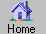Prev Next Index-> contents reference index search external Up-> CppAD Example ListAllExamples CppAD-> Install Introduction AD ADFun preprocessor multi_thread utility ipopt_solve Example speed Appendix Example-> get_started.cpp General ExampleUtility ListAllExamples testvector wno_conversion ListAllExamples Headings

$\newcommand{\W}{ \; #1 \; } \newcommand{\R}{ {\rm #1} } \newcommand{\B}{ {\bf #1} } \newcommand{\D}{ \frac{\partial #1}{\partial #2} } \newcommand{\DD}{ \frac{\partial^2 #1}{\partial #2 \partial #3} } \newcommand{\Dpow}{ \frac{\partial^{#1}}{\partial {#2}^{#1}} } \newcommand{\dpow}{ \frac{ {\rm d}^{#1}}{{\rm d}\, {#2}^{#1}} }$
List All (Except Deprecated) CppAD Examples